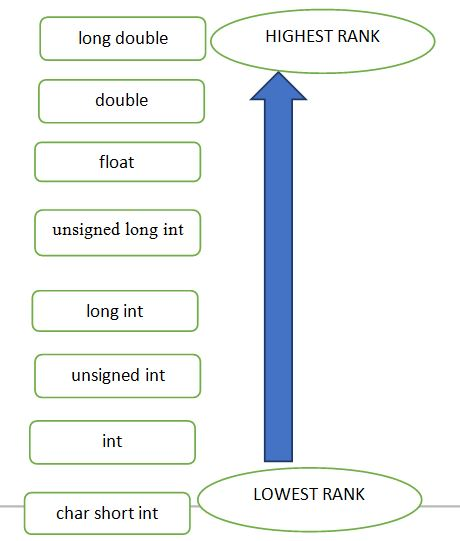# Type Conversion in C

In this article, we are going to learn about Type conversion in C programming language, types of type conversion, their rules etc.
Submitted by Sudarshan Paul, on June 13, 2018

The type conversion process in C is basically converting one type of data type to other to perform some operation. The conversion is done only between those datatypes wherein the conversion is possible ex – char to int and vice versa.

### 1) Implicit Type Conversion

This type of conversion is usually performed by the compiler when necessary without any commands by the user. Thus it is also called "Automatic Type Conversion".

The compiler usually performs this type of conversion when a particular expression contains more than one data type. In such cases either type promotion or demotion takes place.

Rules...

1. char or short type operands will be converted to int during an operation and the outcome data type will also be int.
2. If an operand of type long double is present in the expression, then the corresponding operand will also be converted to long double same for the double data type.
3. If an operand of float type is present then the corresponding operand in the expression will also be converted to float type and the final result will also be float type.
4. If an operand of unsigned long int is present then the other operand will be converted to unsigned long int and the final result will also be unsigned long int.
5. If an operand of long int is present then the other operand will be converted to long int and the final result will also be long int.
6. If an operand of unsigned int is present then the other operand will be converted to unsigned int and the final result will also be unsigned int.

Now, let’s focus on some examples to further understand about type conversions in C.

Example 1

```    int a = 20;
double b = 20.5;
a + b;
```

Here, first operand is int type and other is of type double. So, as per rule 2, the variable a will be converted to double. Therefore, the final answer is double a + b = 40.500000.

Example 2

```    char ch='a';
int a =13;

a + c;
```

Here, first operand is char type and other is of type int. So, as per rule 1, the char variable will be converted to int type during the operation and the final answer will be of type int. We know the ASCII value for ch is 97. Therefore, final answer is a + c = 97 + 13 = 110.

Example 3

```    char ch='A';
unsigned int a =60;

a * b;
```

Here, the first operand is char type and the other is of type unsigned int. So, as per rule 6, char data type will be converted to unsigned int during the operation. Therefore, the final result will be an unsigned int variable, 65 + 60 = 125.

Thus, all these above illustrations suggest that whenever the compiler deals with different data types in an expression, the operand which is present at the lower rank will be converted to the corresponding datatype of the operand with the higher rank.

For ease of understanding follow the flowchart given below, in which datatypes have been arranged in a hierarchy of highest to the lowest rank.### 2) Explicit Type Conversion

Explicit type conversion rules out the use of compiler for converting one data type to another instead the user explicitly defines within the program the datatype of the operands in the expression.

The example below illustrates how explicit conversion is done by the user.

Example:

```double da = 4.5;
double db = 4.6;
double dc = 4.9;

//explicitly defined by user
int result = (int)da + (int)db + (int)dc;

printf("result = %d", result);
```

Output

```    result = 12
```

Thus, in the above example we find that the output result is 12 because in the result expression the user has explicitly defined the operands (variables) as integer data type. Hence, there is no implicit conversion of data type by the compiler.

If in case implicit conversion was used the result would be 13.